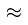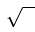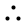# Mathematical Symbols

Symbols save time and space when writing. Here are the most common mathematical symbols:

Symbol Meaning Example
subtract 5−2 = 3
× multiply 4×3 = 12
÷ divide 20÷5 = 4
/ divide 20/5 = 4
( ) grouping symbols 2(a−3)
[ ] grouping symbols 2[ a−3(b+c) ]
{ } set symbols {1, 2, 3}
π pi A = πr2
infinity ∞ is endless
= equals 1+1 = 2approximately equal to π3.14
not equal to π 2
< ≤ less than, less than or equal to 2 < 3
> ≥ greater than, greater than or equal to 5 > 1square root ("radical") √4 = 2
° degrees 20°therefore a=bb=a